# 算法基础-非线性结构

## 有根树

### 二叉树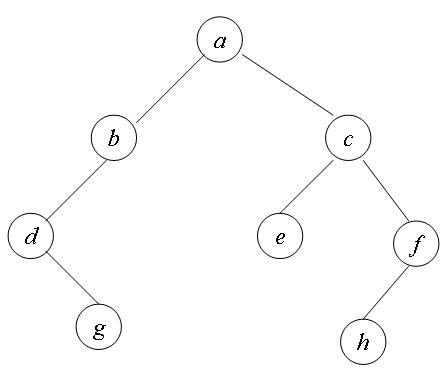### 多叉树

struct Node;
struct Tree;

struct Node{
Tree* tree;
Node* next;
};

struct Tree{
int value;
Node* child;
};

### 树的遍历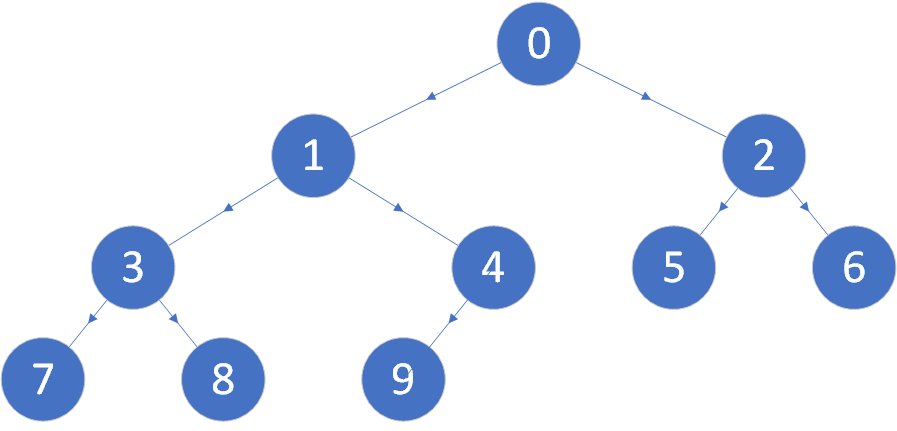#### 深度优先遍历

void DFS(Tree* tree){
if(tree != NULL){
DFS(tree->lChild);
printf("%d ",tree->value);
DFS(tree->rChild);
}
}

#### 广度优先遍历

void BFS(Tree* tree){
if(tree == NULL) return;
queue<Tree*>* q = new queue<Tree*>();
q->push(tree);
while (!q->empty()){
Tree* front = q->front();
q->pop();
printf("%d ",front->value);
if(front->lChild != NULL) q->push(front->lChild);
if(front->rChild != NULL) q->push(front->rChild);
free(front);
}
}

## 图

### 矩阵法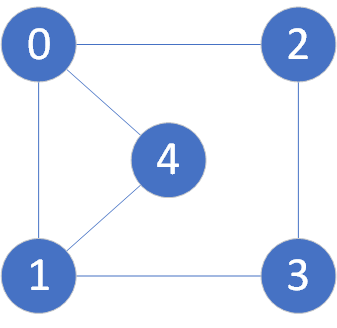5阶矩阵M
01234
011101
111011
210110
301110
411001

### 邻接链表法

struct Vertex{
int value;
Edge* edge;
};

struct Edge{
Vertex* vertex;
Edge* next;
};

### 图的遍历

struct Vertex{
int value;
bool isVisited;
Edge* edge;
};

struct Edge{
Vertex* vertex;
Edge* next;
};

#### 深度优先遍历

void DFS(Vertex* vertex){
if(vertex != NULL){
printf("%d ",vertex->value);
vertex->isVisited = true;
}
Edge* edge = vertex->edge;
while (edge != NULL){
if(!edge->vertex->isVisited){
DFS(edge->vertex);
}
edge = edge->next;
}
}

#### 广度优先遍历

void BFS(Vertex* vertex){
queue<Vertex*> q;
q.push(vertex);
while (!q.empty()){
Vertex* front = q.front();
printf("%d ",front->value);
front->isVisited = true;
q.pop();
Edge* edge = front->edge;
while (edge != NULL){
if(!edge->vertex->isVisited){
q.push(edge->vertex);
edge->vertex->isVisited = true;
}
edge = edge->next;
}
}
}

### 拓扑序列

#### 拓扑排序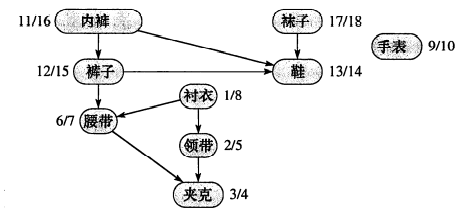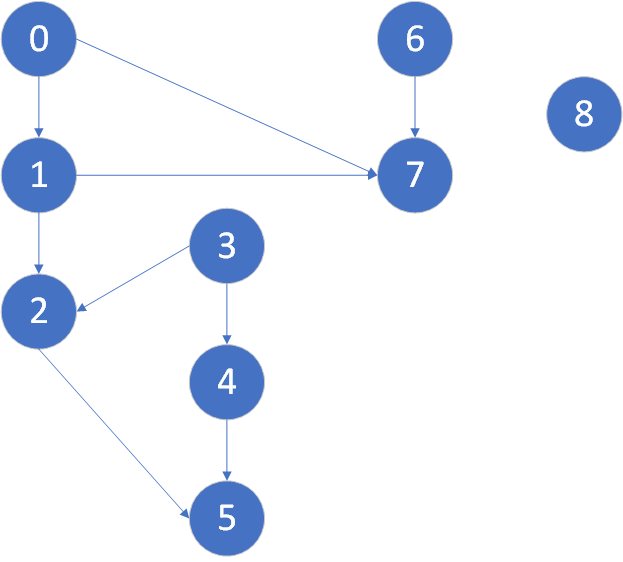8,6,3,4,0,1,7,2,5

struct Vertex{
int index;
int startTime;
int endTime;
bool isVisited;
Edge* edge;
};

struct Edge{
Vertex* vertex;
Edge* next;
};

class Graph{
public:
int size;
Graph(int size);
Vertex* get(int index);
void GetDFSTime();

private:
Vertex** G;
void DFS(int index, int* order);
};

下面是具体实现代码

Graph::Graph(int size) {
this->size = size;
G = (Vertex**) malloc(size * sizeof(Vertex));
for(int i=0;i<size;i++){
G[i] = (Vertex*)malloc(sizeof(Vertex));
G[i]->index = i;
G[i]->isVisited = false;
G[i]->edge = NULL;
}
}

void Graph::addEdge(int edge1, int edge2) {
Edge* edge = (Edge*)malloc(sizeof(Edge));
edge->vertex = G[edge2];
edge->next = NULL;
if(G[edge1]->edge == NULL){
G[edge1]->edge = edge;
}else{
Edge* e = G[edge1]->edge;
while (e->next != NULL){e = e->next;}
e->next = edge;
}
}

Vertex* Graph::get(int index) {
if(index < 0 || index >= size){
return NULL;
}else{
return G[index];
}
}

void Graph::GetDFSTime(){
int order = 0;
for(int i=0;i<size;i++){
DFS(i,&order);
}
for(int i=0;i<size;i++){
printf("index =%2d: %2d, %2d\n",G[i]->index, G[i]->startTime, G[i]->endTime);
}
}

void Graph::DFS(int index, int* order){
Vertex* vertex = get(index);
if(vertex == NULL || vertex->isVisited) return;
G[index]->startTime = ++(*order);
vertex->isVisited = true;
Edge* edge = vertex->edge;
while (edge != NULL){
if(!edge->vertex->isVisited){
DFS(edge->vertex->index, order);
}
edge = edge->next;
}
G[index]->endTime = ++(*order);
}

进行测试

int main()
{
Graph g(9);
g.GetDFSTime();
}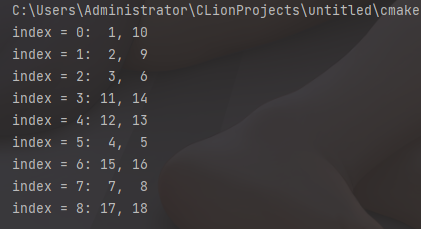#### DFS与拓扑序列的关系

08-264438
06-306万+
08-202280
03-16550
04-156321
03-246056
10-26241
11-141646
08-255万+
04-091943
03-022万+
10-301039
10-191万+

### “相关推荐”对你有帮助么？

•非常没帮助
•没帮助
•一般
•有帮助
•非常有帮助被折叠的  条评论 为什么被折叠?到【灌水乐园】发言Dear_Xuan

¥1 ¥2 ¥4 ¥6 ¥10 ¥20获取中扫码支付点击重新获取扫码支付1.余额是钱包充值的虚拟货币，按照1:1的比例进行支付金额的抵扣。
2.余额无法直接购买下载，可以购买VIP、付费专栏及课程。余额充值